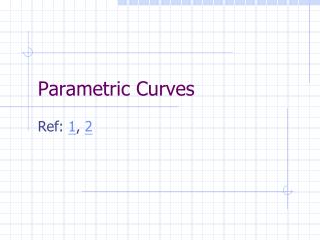# Parametric Curves - PowerPoint PPT PresentationDownload PresentationParametric Curves

Parametric CurvesDownload Presentation## Parametric Curves

- - - - - - - - - - - - - - - - - - - - - - - - - - - E N D - - - - - - - - - - - - - - - - - - - - - - - - - - -
##### Presentation Transcript

1. Parametric Curves Ref: 1, 2

2. Outline • Hermite curves • Bezier curves • Catmull-Rom splines • Frames along the curve

3. Hermite Curves • 3D curve of polynomial bases • Geometrically defined by position and tangents of end points • Able to construct C1 composite curve • In CG, often used as the trace for camera with Frenet frame, or rotation-minimizing frame

4. h1(s) = 2s3 - 3s2 + 1 h2(s) = -2s3 + 3s2 h3(s) = s3 - 2s2 + s h4(s) = s3 - s2 P(s) = P1h1(s) + P2h2(s) + T1h3(s) + T2h4(s) P’(s)=P1h1’(s) + P2h2’(s) + T1h3’(s) + T2h4’(s) h1’ = 6s2-6s h2’ = -6s2+6s h3’= 3s2-4s+1 h4’= 3s2– 2s Math … P(0)= P1, P(1)=P2; P’(0)=T1, P’(1)=T2

5. Blending Functions • At s = 0: • h1 = 1, h2 = h3 = h4 = 0 • h1’ = h2’ = h4’ = 0, h3’ = 1 • At s = 1: • h1 = h3 = h4 = 0, h2 = 1 • h1’ = h2’ = h3’ = 0, h4’ = 1 h2(s) h4(s) h3(s) h1(s) P(0) = P1 P’(0) = T1 P(1) = P2 P’(1) = T2

6. C1 Composite Curve P(t) Q(t) R(t) More on Continuity

7. t1 t3 P(t) Q(t) R(t) t2 t0 Composite Curve Each subcurve is defined in [0,1]. The whole curve (PQR) can be defined from [0,3] To evaluate the position (and tangent)

8. Close Relatives Bezier curves Catmull-Rom splines

9. Defined by four control points de Casteljau algorithm (engineer at Citroën) Bezier Curve (cubic, ref)

10. Also invented by Pierre Bézier (engineer of Renault) Blending function: Bernstein polynomial Can be of any degree Bezier Curve (cont) Degree n has (n+1) control points

11. First Derivative of Bezier Curves (ref) • Degree-n Bezier curve • Bernstein polynomial • Derivative of Bernstein polynomial • First derivative of Bezier curve • Hodograph

12. Ex: cubic Bezier curve Hence, to convert to/from Hermite curve:

13. C1 Composite Bezier Curves

14. Bezier Curve Fitting • From Graphics Gems (code) • Input: digitized data points in R2 • Output: composite Bezier curves in specified error

15. Bezier Marching • A path made of composite Bezier curves • Generate a sequence of points along the path with nearly constant step size • Adjust the parametric increment according to (approximated) arc length

16. Catmull-Rom spline (1974, ref) • Given n+1 control points {P0,…,Pn}, we wish to find a curve that interpolates these control points (i.e. passes through them all), and is local in nature (i.e. if one of the control points is moved, it only affects the curve locally). • We define the curve on each segment [Pi,Pi+1] by using the two control points, and specifying the tangent to the curve at each control point to be (Pi+1–Pi-1)/2 and (Pi+2–Pi)/2 • Tangents in first and last segments are defined differently

17. Gives you a Catmull-Rom spline, open or close. PowerPoint Line Tool …

18. Ex: Catmull-Rom Curves

19. Reference Frames Along the Curve • Applications • generalized cylinder • Cinematography • Frenet frames • Rotation minimizing frame

20. Generalized Cylinder

21. tangent vector binormal vector main normal vector Frenet Frame (Farin) : cross product Unit vectors

22. Frenet Frame (arc-length parameterization)

23. In this notation, the curve is r(s) Express T’N’B’ (change rate of TNB) in terms of TNB Frenet-Serret Formula    Orthonormal expansion

24. Frenet-Serret Formula (cont) In general parameterization r(t) Curvature and torsion r(t)=(x(t),y(t))

25. (s) Geometric Meaning of k and t curvature torsion x(s+Ds) Da: angle between t(s) and t(s+Ds) Db: angle between b(s) and b(s+Ds) More result on this reference

26. Frenet Frame Problem Problem: vanishing second derivative at inflection points (vanishing normal)

27. Use the second derivative to define the first frame (if zero, set N0 to any vector T0) Compute all subsequent Ti; find a rotation from Ti-1 to Ti; rotate Ni and Bi accordingly If no rotation, use the same frame Rotation Minimizing Frame (ref)

28. Continuity Geometric Continuity • A curve can be described as having Gn continuity, n being the increasing measure of smoothness. • G0: The curves touch at the join point. • G1: The curves also share a common tangent direction at the join point. • G2: The curves also share a common center of curvature at the join point. Parametric Continuity • C0: curves are joined • C1: first derivatives are equal • C2: first and second derivatives are equal • Cn: first through nth derivatives are equal BACK